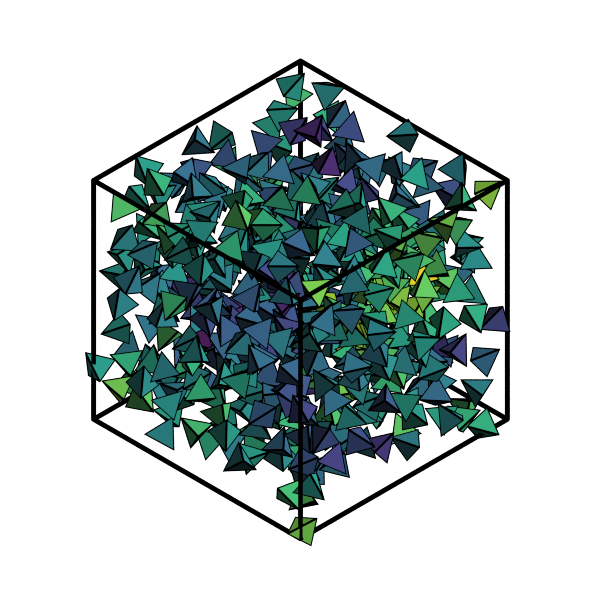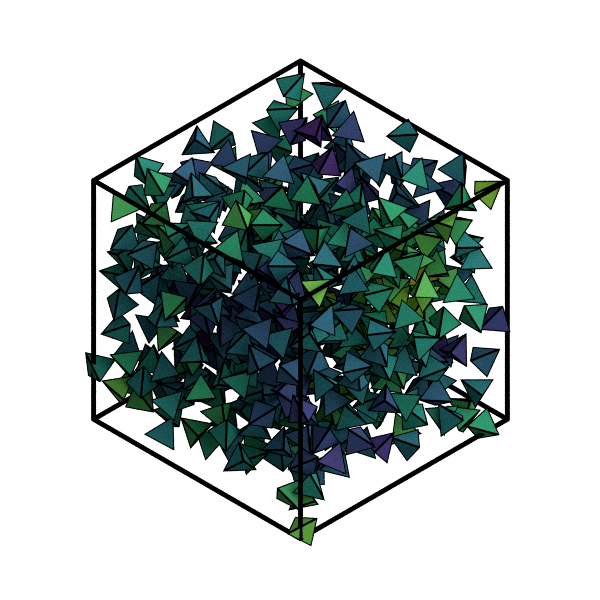# Visualizing analyses with fresnel¶

In this notebook, we simulate a system of tetrahedra, color particles according to their local density, and path-trace the resulting image with fresnel.

The cell below runs a short HOOMD-blue simulation of tetrahedra using Hard Particle Monte Carlo (HPMC).

:

import hoomd
import hoomd.hpmc
hoomd.context.initialize('')

# Create an 8x8x8 simple cubic lattice
system = hoomd.init.create_lattice(
unitcell=hoomd.lattice.sc(a=1.5), n=8)

# Create our tetrahedra and configure the HPMC integrator
mc = hoomd.hpmc.integrate.convex_polyhedron(seed=42)
mc.set_params(d=0.2, a=0.1)
vertices = [( 0.5, 0.5, 0.5),
(-0.5,-0.5, 0.5),
(-0.5, 0.5,-0.5),
( 0.5,-0.5,-0.5)]
mc.shape_param.set('A', vertices=vertices)

# Run for 5,000 steps
hoomd.run(5e3)
snap = system.take_snapshot()

HOOMD-blue v2.6.0-7-g60513d253 DOUBLE HPMC_MIXED MPI TBB SSE SSE2 SSE3 SSE4_1 SSE4_2 AVX AVX2
Compiled: 06/13/2019
Copyright (c) 2009-2019 The Regents of the University of Michigan.
-----
You are using HOOMD-blue. Please cite the following:
* J A Anderson, C D Lorenz, and A Travesset. "General purpose molecular dynamics
simulations fully implemented on graphics processing units", Journal of
Computational Physics 227 (2008) 5342--5359
* J Glaser, T D Nguyen, J A Anderson, P Liu, F Spiga, J A Millan, D C Morse, and
S C Glotzer. "Strong scaling of general-purpose molecular dynamics simulations
on GPUs", Computer Physics Communications 192 (2015) 97--107
-----
-----
You are using HPMC. Please cite the following:
* J A Anderson, M E Irrgang, and S C Glotzer. "Scalable Metropolis Monte Carlo
for simulation of hard shapes", Computer Physics Communications 204 (2016) 21
--30
-----
HOOMD-blue is running on the CPU
notice(2): Group "all" created containing 512 particles
** starting run **
Time 00:00:10 | Step 2053 / 5000 | TPS 205.218 | ETA 00:00:14
Time 00:00:20 | Step 4159 / 5000 | TPS 210.543 | ETA 00:00:03
Time 00:00:24 | Step 5000 / 5000 | TPS 210.467 | ETA 00:00:00
Average TPS: 208.306
---------
notice(2): -- HPMC stats:
notice(2): Average translate acceptance: 0.749166
notice(2): Average rotate acceptance:    0.867601
notice(2): Trial moves per second:        426589
notice(2): Overlap checks per second:     3.06914e+07
notice(2): Overlap checks per trial move: 71.946
notice(2): Number of overlap errors:      0
** run complete **


Now we import the modules needed for analysis and visualization.

:

import fresnel
import freud
import matplotlib.cm
from matplotlib.colors import Normalize
import numpy as np
device = fresnel.Device()


Next, we’ll set up the arrays needed for the scene and its geometry. This includes the analysis used for coloring particles.

:

poly_info = fresnel.util.convex_polyhedron_from_vertices(vertices)
positions = snap.particles.position
orientations = snap.particles.orientation
box = freud.box.Box.from_box(snap.box)
ld = freud.density.LocalDensity(3.0, 1.0, 1.0)
ld.compute(box, positions)
colors = matplotlib.cm.viridis(Normalize()(ld.density))
box_points = np.asarray([
box.makeCoordinates(
[[0, 0, 0], [0, 0, 0], [0, 0, 0], [1, 1, 0],
[1, 1, 0], [1, 1, 0], [0, 1, 1], [0, 1, 1],
[0, 1, 1], [1, 0, 1], [1, 0, 1], [1, 0, 1]]),
box.makeCoordinates(
[[1, 0, 0], [0, 1, 0], [0, 0, 1], [1, 0, 0],
[0, 1, 0], [1, 1, 1], [1, 1, 1], [0, 1, 0],
[0, 0, 1], [0, 0, 1], [1, 1, 1], [1, 0, 0]])])


This cell creates the scene and geometry objects to be rendered by fresnel.

:

scene = fresnel.Scene(device)
geometry = fresnel.geometry.ConvexPolyhedron(
scene, poly_info,
position=positions,
orientation=orientations,
color=fresnel.color.linear(colors))
geometry.material = fresnel.material.Material(
color=fresnel.color.linear([0.25, 0.5, 0.9]),
roughness=0.8, primitive_color_mix=1.0)
geometry.outline_width = 0.05
box_geometry = fresnel.geometry.Cylinder(
scene, points=box_points.swapaxes(0, 1))
box_geometry.color[:] = np.tile([0, 0, 0], (12, 2, 1))
box_geometry.material.primitive_color_mix = 1.0
scene.camera = fresnel.camera.fit(scene, view='isometric', margin=0.1)


First, we preview the scene. (This doesn’t use path tracing, and is much faster.)

:

fresnel.preview(scene, aa_level=3, w=600, h=600)

:Finally, we use path tracing for a high quality image. The number of light samples can be increased to reduce path tracing noise.

:

fresnel.pathtrace(scene, light_samples=16, w=600, h=600)

: